# Test: Continuity

## 10 Questions MCQ Test Mathematics (Maths) Class 12 | Test: Continuity

Description
This mock test of Test: Continuity for JEE helps you for every JEE entrance exam. This contains 10 Multiple Choice Questions for JEE Test: Continuity (mcq) to study with solutions a complete question bank. The solved questions answers in this Test: Continuity quiz give you a good mix of easy questions and tough questions. JEE students definitely take this Test: Continuity exercise for a better result in the exam. You can find other Test: Continuity extra questions, long questions & short questions for JEE on EduRev as well by searching above.
QUESTION: 1

### Which of the following functions are not continuous.

Solution:

Here, f(x)=[X] could be expressed graphically as: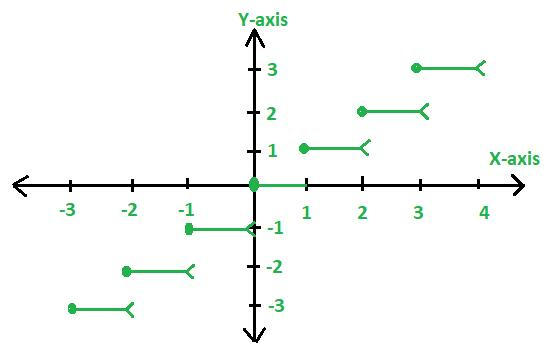From the graph it is clear that the function is discontinuous.

QUESTION: 2

### If f (x) = [xsinπx] { where [x] denotes greatest integer function}, then f (x) is

Solution:

► By the definition of [x], we have f(x)=[xsinπx]=0 for −1≤x≤1, because 0 ≤ xsin(πx) ≤1

Also, f(x)=[xsinπx]=−1, for 1<x<1+h for some small appropriate h> 0, because sinπx is negative and ≥−1 for 1<x<1+h.

Thus f(x) is constant and equal to 0 in the interval [−1;1] and so it is continuous and differentiable in (−1,1).

In particular, f(x) is continuous at x=0

QUESTION: 3

### Examine the continuity of the function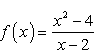Solution:

f’(x) = (x2-4)/(x-2)

= [(x-2)(x+2)]/(x-2)

= (x+2) which is continuous everywhere.

QUESTION: 4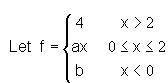For what values of a and b, f is a continuous function.

Solution:
QUESTION: 5

Discuss the continuity of function  f(x) = |x-1| + |x+1|, x R

Solution:

f(x) = |x-1| + |x+1| , x € R

► For x ≥ 1,
f(x) = x-1 + x+1
f(x) = 2x

► For -1 ≤ x ≤ 1,
f(x) = -x + 1 + x+1
f(x) = 2

► For x ≤ -1,
f(x) = -x + 1 - x-1
f(x) = -2x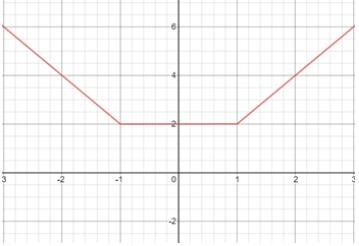As the graph of f(x) shows, the function is continuous throughout its domain.

QUESTION: 6

Examine the continuity of function  f(x) = (x-1)(x-2)

Solution:

Lim f (x) = lim (x-1)(x-2)  at x tend to k

► So it get    k2-3k+2

► Now f (k) = k2 -3k+2

► So f (x) =f (k) so continous at everywhere

QUESTION: 7

What is the point of discontinuity for signum function?

Solution:

Sgn(x)=|x|/x
► for x>0, sgn(x)=1
► for x=0, sgn(x)=0
► for x<0, sgn(x)="">
► Now see graphically or theoretically sgn(x) is discontinuous at x=0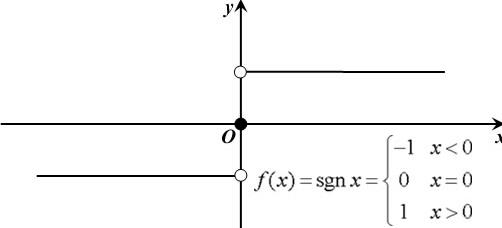QUESTION: 8

Function f(x) = log x +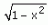is continuous at​

Solution:

[-1,1] cannot be continuous interval because log is not defined at 0

The value of x cannot be greater than 1 because then the function will become complex

(0,1) will not be considered because its continuous at 1 as well. Hence D is the correct option

QUESTION: 9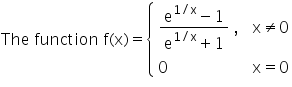Solution: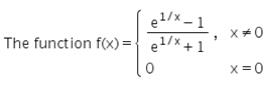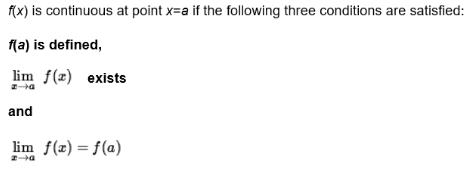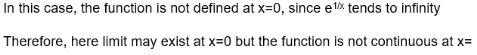Function is not continuous at x=0

QUESTION: 10

A real function f is said to be continuous if it is continuous at every point in …… .​

Solution: